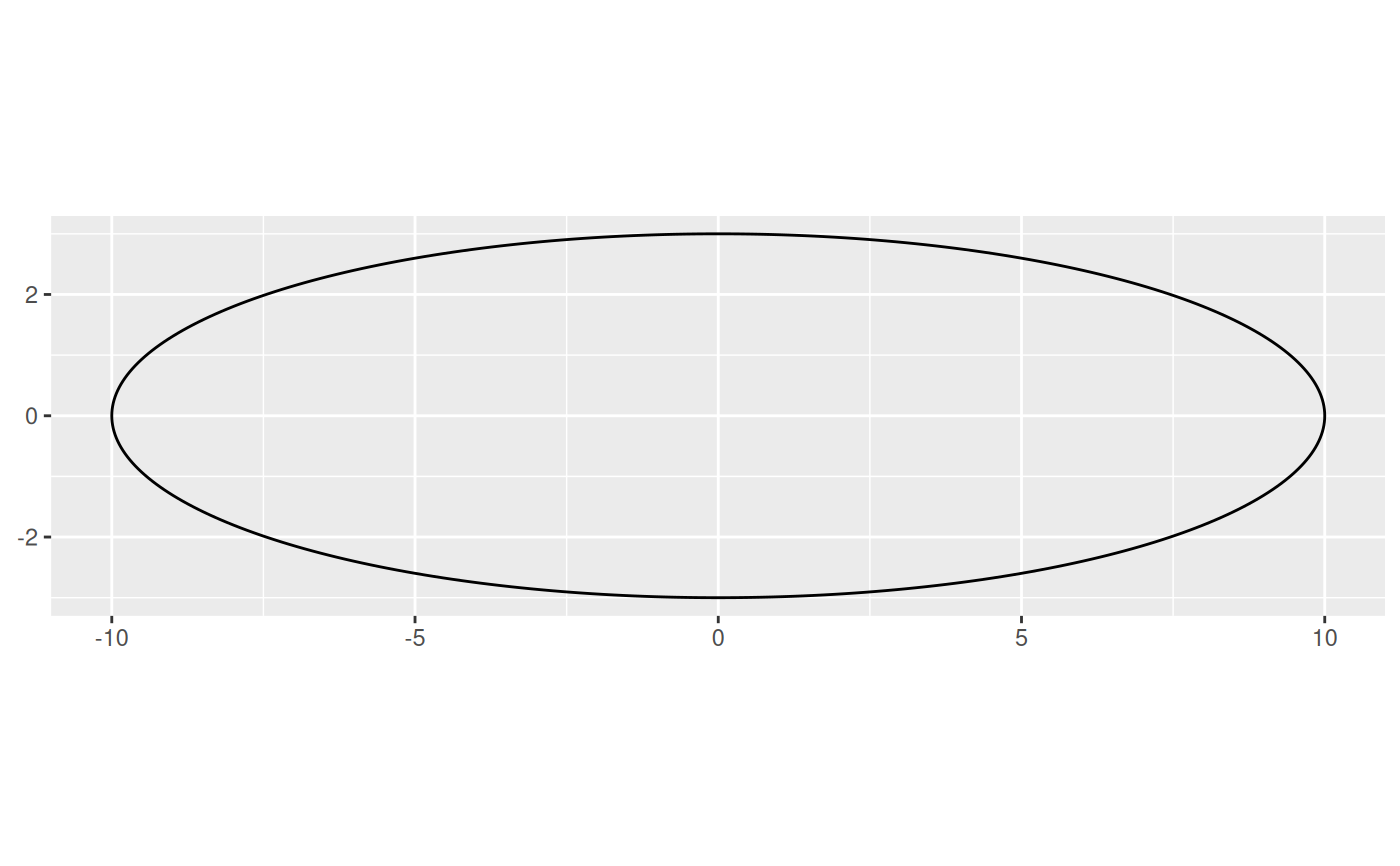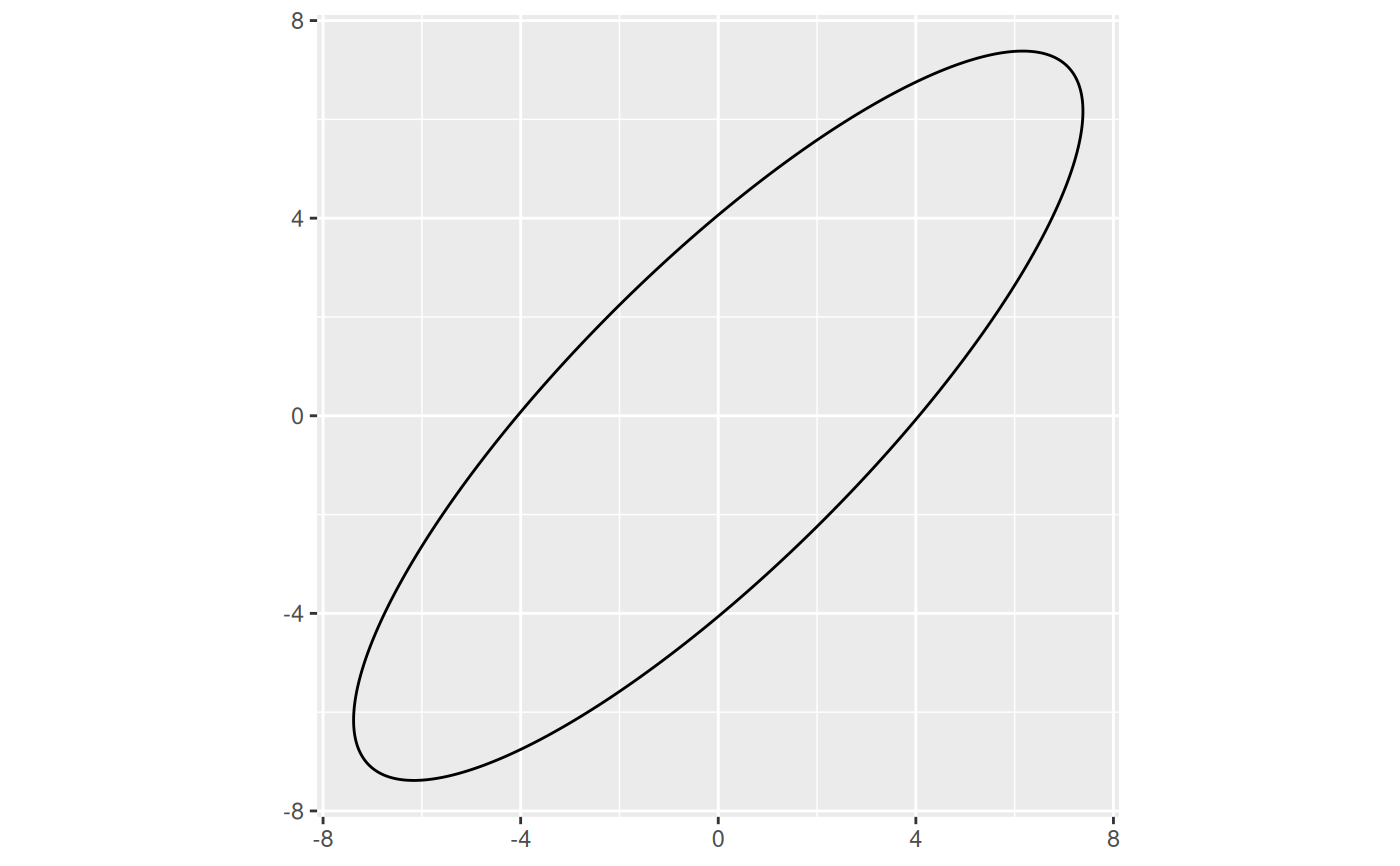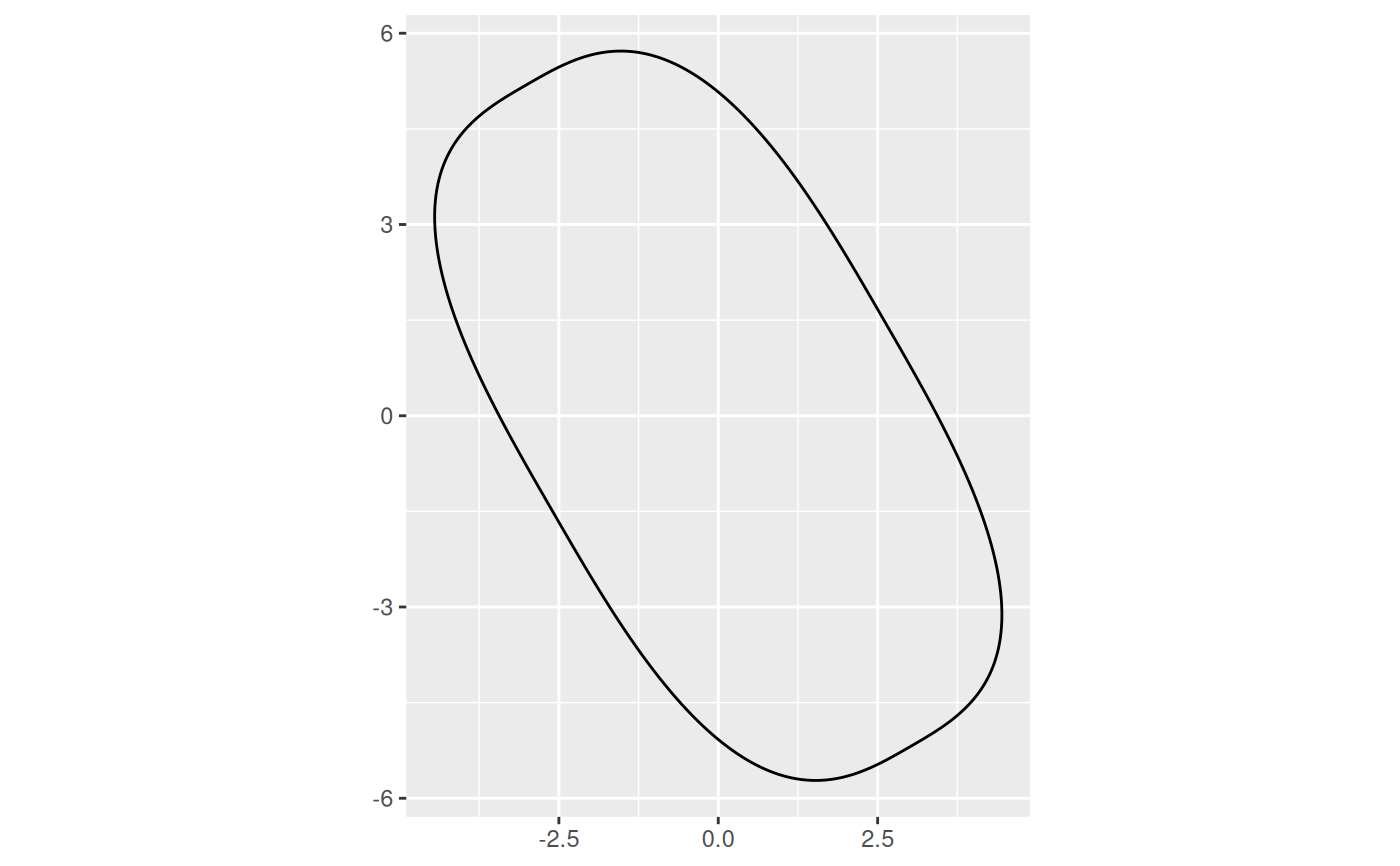This is a generalisation of geom_circle() that allows you to draw ellipses at a specified angle and center relative to the coordinate system. Apart from letting you draw regular ellipsis, the stat is using the generalised formula for superellipses which can be utilised by setting the m1 and m2 aesthetics. If you only set the m1 the m2 value will follow that to ensure a symmetric appearance.

stat_ellip(
mapping = NULL,
data = NULL,
geom = "circle",
position = "identity",
n = 360,
na.rm = FALSE,
show.legend = NA,
inherit.aes = TRUE,
...
)

geom_ellipse(
mapping = NULL,
data = NULL,
stat = "ellip",
position = "identity",
n = 360,
na.rm = FALSE,
show.legend = NA,
inherit.aes = TRUE,
...
)

## Arguments

mapping

Set of aesthetic mappings created by aes(). If specified and inherit.aes = TRUE (the default), it is combined with the default mapping at the top level of the plot. You must supply mapping if there is no plot mapping.

data

The data to be displayed in this layer. There are three options:

If NULL, the default, the data is inherited from the plot data as specified in the call to ggplot().

A data.frame, or other object, will override the plot data. All objects will be fortified to produce a data frame. See fortify() for which variables will be created.

A function will be called with a single argument, the plot data. The return value must be a data.frame, and will be used as the layer data. A function can be created from a formula (e.g. ~ head(.x, 10)).

geom

The geometric object to use to display the data, either as a ggproto Geom subclass or as a string naming the geom stripped of the geom_ prefix (e.g. "point" rather than "geom_point")

position

Position adjustment, either as a string naming the adjustment (e.g. "jitter" to use position_jitter), or the result of a call to a position adjustment function. Use the latter if you need to change the settings of the adjustment.

n

The number of points to sample along the ellipse.

na.rm

If FALSE, the default, missing values are removed with a warning. If TRUE, missing values are silently removed.

show.legend

logical. Should this layer be included in the legends? NA, the default, includes if any aesthetics are mapped. FALSE never includes, and TRUE always includes. It can also be a named logical vector to finely select the aesthetics to display.

inherit.aes

If FALSE, overrides the default aesthetics, rather than combining with them. This is most useful for helper functions that define both data and aesthetics and shouldn't inherit behaviour from the default plot specification, e.g. borders().

...

Other arguments passed on to layer(). These are often aesthetics, used to set an aesthetic to a fixed value, like colour = "red" or size = 3. They may also be parameters to the paired geom/stat.

stat

The statistical transformation to use on the data for this layer, either as a ggproto Geom subclass or as a string naming the stat stripped of the stat_ prefix (e.g. "count" rather than "stat_count")

## Aesthetics

geom_arc understand the following aesthetics (required aesthetics are in bold):

• x0

• y0

• a

• b

• angle

• m1

• m2

• color

• fill

• linewidth

• linetype

• alpha

• lineend

## Computed variables

x, y

The coordinates for the points along the ellipse

## Examples

# Basic usage
ggplot() +
geom_ellipse(aes(x0 = 0, y0 = 0, a = 10, b = 3, angle = 0)) +
coord_fixed()# Rotation
# Note that it expects radians and rotates the ellipse counter-clockwise
ggplot() +
geom_ellipse(aes(x0 = 0, y0 = 0, a = 10, b = 3, angle = pi / 4)) +
coord_fixed()# Draw a super ellipse
ggplot() +
geom_ellipse(aes(x0 = 0, y0 = 0, a = 6, b = 3, angle = -pi / 3, m1 = 3)) +
coord_fixed()